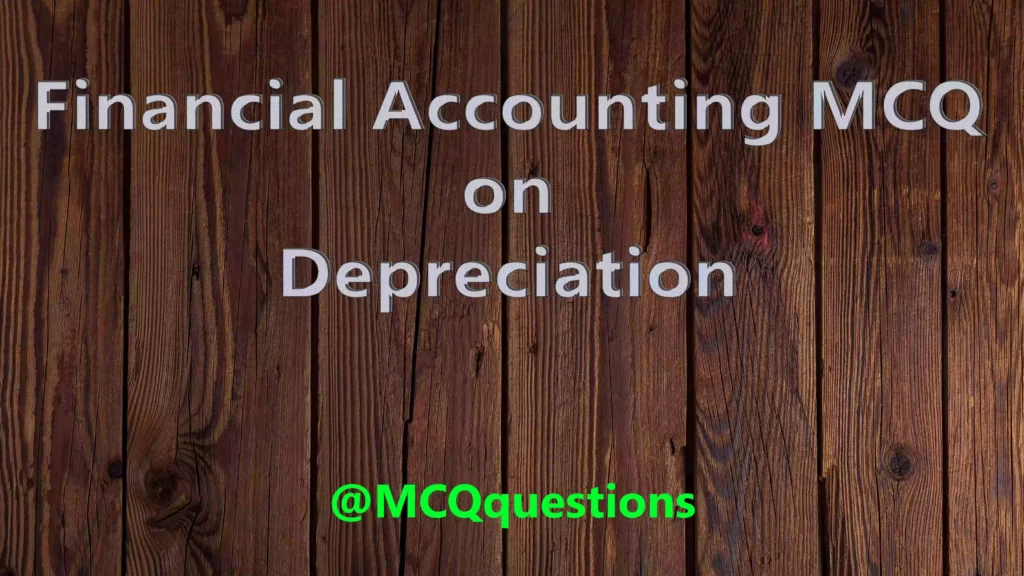# Financial Accounting MCQ on Depreciation

## MCQ on Depreciation

1. Erosion, rust, rot, and decay cause of depreciation is an example of

(a) Physical deterioration

(b) Economic factor

(c) Time factors

(d) None of the above

`Answer (a) Physical deterioration`

2. Lease, patents and copy right related to which cause of depreciation

(a) Physical deterioration

(b) Economic factor

(c) Time factors

(d) None of the above

`Answer (c) Time factors`

3. Obsolescence means is

(a) Update

(b) Out of date

(c) Up to date

(d) None of the above

`Answer (b) Out of date`

4. Decrease in the value of natural assets is

(a) Depreciation

(b) Depletion

(c) Amortization

(d) None of the above

`Answer (b) Depletion`

5. Decrease in the value of fixed assets is

(a) Depletion

(b) Amortization

(c) Depreciation

(d) None of the above

`Answer (c) Depreciation`

6. Decrease in the value of intangible assets is

(a) Amortization

(b) Depreciation

(c) Depletion

(d) None of the above

`Answer (a) Amortization`

7. Depreciation is a process of

(a) Allocation

(b) Valuation

(c) Both

(d) None of the above

`Answer (a) Allocation`

8. Depreciation arise because due to

(a) Physical wear and tear

(b) Fall in money value

(c) Fall in market price

(d) None of the above

`Answer (a) Physical wear and tear`

9. Under straight line methods of charging depreciation, value of depreciation

(a) Increase every year

(b) Decrease every year

(c) Constant every year

(d) None of the above

`Answer (c) Constant every year`

10. Under Diminishing Balance methods of charging depreciation, value of depreciation

(a) Constant every year

(b) Decrease every year

(c) Increase every year

(d) None of the above

`Answer (b) Decrease every year`

11. Under straight line methods of charging depreciation, value of depreciation charge on

(a) Original cost

(b) Written down value

(c) Scrap value

(d) None of the above

`Answer  (a) Original cost`

12. Under Diminishing Balance methods of charging depreciation, value of depreciation charge on

(a) Originalcost

(b) Scrap value

(c) Written down value

(d) None of the above

`Answer (c) Written down value`

13. Value of assets may be reduce to zero in which methods, if depreciation charge throughout the life of the assets

(a) Fixed installment methods

(b) Written down value methods

(c) Insurance policy methods

(d) None of the above

`Answer (a) Fixed installment methods`

14. The amount of depreciation charge on machinery will be debited to

(a) Machinery account

(b) Cash account

(c) Depreciation account

(d) None of the above

`Answer (c) Depreciation account`

15. Loss on sale of machinery should be written off against

(a) Assets account

(b) Depreciation fund account

(c) Sale account

(d) Loss account

`Answer (b) Depreciation fund account`

16. Depreciation is ……..of cost of fixed assets

(a) Apportionment

(b) Allocation

(c) Expenses

(d) None of the above

`Answer  (a) Apportionment`

17. Mines assets related to

(a) Depreciation

(b) Amortization

(c) Depletion

(d) None of the above

`Answer (c) Depletion`

18. The term refer to the damage done to a building or other property during tenancy is called as

(a) Dilapidation

(b) Depreciation

(c) Depletion

(d) All of the above

`Answer (a) Dilapidation`

19. Why depreciation is charged

(a) To know the true profit

(b) To make provision for replacement

(c) To show true financial position

(d) All of the above

`Answer (d) All of the above`

20. What is the cause of depreciation?

(a) Rot & Rust

(b) Out of date

(c) Extraction of oil from oil well

(d) All of the above

`Answer (d) All of the above`